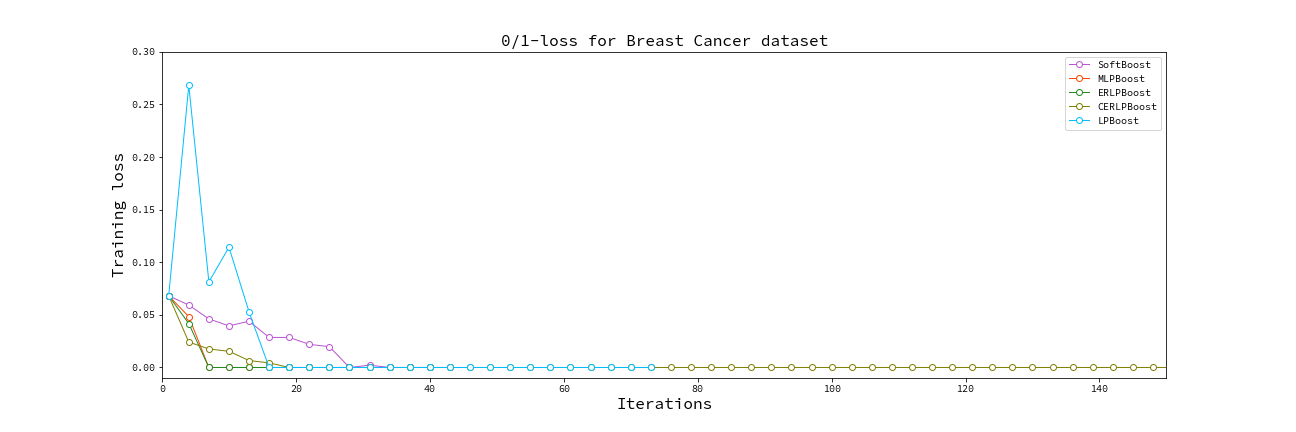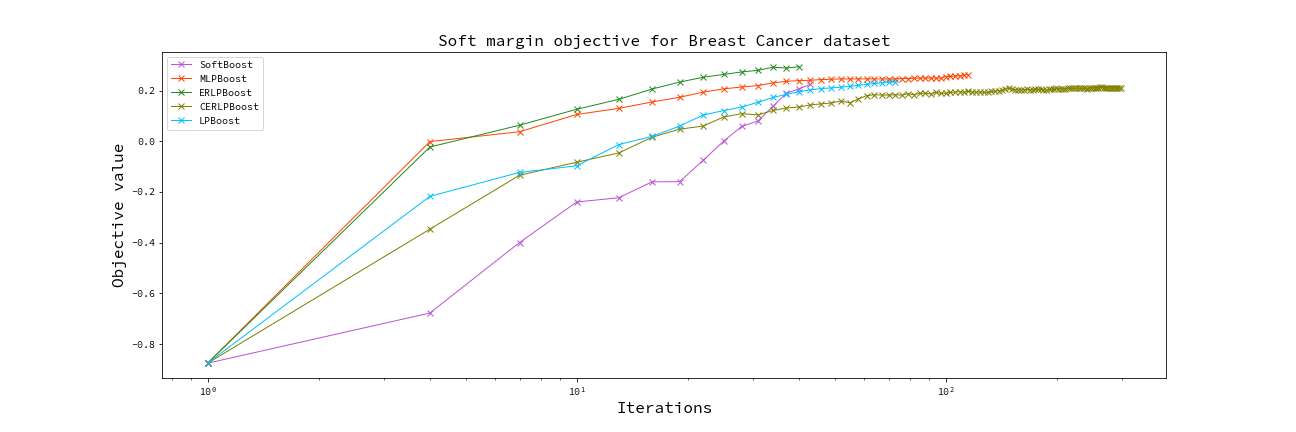## miniboosts

MiniBoosts: A collection of boosting algorithms written in Rust 🦀

### 2 unstable releases

 0.2.0 Mar 13, 2023 Feb 11, 2023

#39 in Machine learning

260KB
6K SLoC

# MiniBoosts

A collection of boosting algorithms written in Rust 🦀.Some boosting algorithms use Gurobi optimizer, so you must acquire a license to use this library. If you have the license, you can use these boosting algorithms (boosters) by specifying `features = ["extended"]` in `Cargo.toml`.

Note! If you are trying to use the `extended` feature without a Gurobi license, the compilation fails.

## Features

Currently, I implemented the following Boosters and Weak Learners.

If you invent a new boosting algorithm, you can introduce it by implementing `Booster` trait. See `cargo doc --open` for details.

### Boosters

`BOOSTER` `FEATURE FLAG`
by Freund and Schapire, 1997
by Rätsch and Warmuth, 2005
SmoothBoost
by Rocco A. Servedio, 2003
TotalBoost
by Warmuth, Liao, and Rätsch, 2006
`extended`
LPBoost
by Demiriz, Bennett, and Shawe-Taylor, 2002
`extended`
SoftBoost
by Warmuth, Glocer, and Rätsch, 2007
`extended`
ERLPBoost
by Warmuth and Glocer, and Vishwanathan, 2008
`extended`
CERLPBoost (Corrective ERLPBoost)
by Shalev-Shwartz and Singer, 2010
`extended`
MLPBoost
by Mitsuboshi, Hatano, and Takimoto, 2022
`extended`
by Jerome H. Friedman

### Weak Learners

`WEAK LEARNERS`
DTree (Decision Tree)
RTree (Regression Tree)

## Future work

• Boosters

• Weak Learners

• Bag of words
• TF-IDF
• Two-Layer Neural Network
• RBF-Net
• Others

• Parallelization
• LP/QP solver (This work allows you to use `extended` features without a license).

## How to use

You can see the document by `cargo doc --open` command.

You need to write the following line to `Cargo.toml`.

``````miniboosts = { git = "https://github.com/rmitsuboshi/miniboosts" }
``````

If you want to use `extended` features, such as `LPBoost`, specify the option:

``````miniboosts = { git = "https://github.com/rmitsuboshi/miniboosts", features = ["extended"] }
``````

Here is a sample code:

``````use miniboosts::prelude::*;

fn main() {
// Set file name
let file = "/path/to/input/data.csv";

// The column named `class` is corresponds to the labels (targets).
.unwrap()
.set_target("class");

// Set tolerance parameter
let tolerance: f64 = 0.01;

// Initialize Booster
.tolerance(tol); // Set the tolerance parameter.

// Initialize Weak Learner
// For decision tree, the default `max_depth` is `None` so that
// The tree grows extremely large.
let weak_learner = DTree::init(&sample)
.max_depth(2) // Specify the max depth (default is not specified)
.criterion(Criterion::Edge); // Choose the split criterion

// Run boosting algorithm
// Each booster returns a combined hypothesis.
let f = booster.run(&weak_learner);

// Get the batch prediction for all examples in `data`.
let predictions = f.predict_all(&sample);

// You can predict the `i`th instance.
let i = 0_usize;
let prediction = f.predict(&sample, i);
}
``````

If you use boosting for soft margin optimization, initialize booster like this:

``````let n_sample = sample.shape().0;
let nu = n_sample as f64 * 0.2;
let lpboost = LPBoost::init(&sample)
.tolerance(tol)
.nu(nu); // Setting the capping parameter.
``````

Note that the capping parameter must satisfies `1 <= nu && nu <= n_sample`.

## Research feature

When you invent a new boosting algorithm and write a paper, you need to compare it to previous works to show the effectiveness of your one. One way to compare the algorithms is to plot the curve for objective value or train/test loss. This crate can output a CSV file for such values in each step.

Here is an example:

``````use miniboosts::prelude::*;
use miniboosts::research::Logger;
use miniboosts::common::objective_functions::SoftMarginObjective;

// Define a loss function
fn zero_one_loss<H>(sample: &Sample, f: &CombinedHypothesis<H>) -> f64
where H: Classifier
{
let n_sample = sample.shape().0 as f64;

let target = sample.target();

f.predict_all(sample)
.into_iter()
.zip(target.into_iter())
.map(|(fx, &y)| if fx != y as i64 { 1.0 } else { 0.0 })
.sum::<f64>()
/ n_sample
}

fn main() {
let path = "/path/to/train/data.csv";
let train = Sample::from_csv(path, true)
.unwrap()
.set_target("class");

// Set some parameters used later.
let n_sample = train.shape().0 as f64;
let nu = 0.01 * n_sample;

let path = "/path/to/test/data.csv";
let test = Sample::from_csv(path, true)
.unwrap()
.set_target("class");

let booster = LPBoost::init(&train);
let weak_learner = DTree::init(&train)
.max_depth(2)
.criterion(Criterion::Entropy);

let mut logger = Logger::new(
booster, tree, objective, zero_one_loss, &train, &test
);

// Each line of `lpboost.csv` contains the following four information:
// Objective value, Train loss, Test loss, Time per iteration
// The returned value `f` is the combined hypothesis.
let f = logger.run("lpboost.csv");
}
``````

Further, one can log your algorithm by implementing `Research` trait.

Run `cargo doc --open` to see more information.

~13MB
~269K SLoC